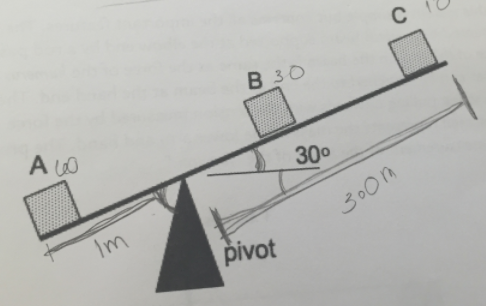# Problem: On a seesaw shown below, mass A is 60 kg, mass B is 30 kg, and mass C is 10 kg. Mass A is 1.0 m from the pivot. Mass C is 3.0 m from the pivot. The seesaw is at an angle of 30° from the horizontal. Part 1: Calculate the torques of mass A and mass C about the pivot. Part2: Where should you place mass B in relation to the pivot for the system to be in rotational equilibrium?

###### FREE Expert Solution

Part 1: Torques created by masses A and C

Torque:

τ = rFsinθ, where θ is the angle between r and F.

We'll take counterclockwise as positive.

τA = rA(mAg)sinθ = (1.0)(60)(9.8)sin60 = 509.2N•m

The torque of mass A about the pivot is 509.2N•m

91% (361 ratings)###### Problem Details

On a seesaw shown below, mass A is 60 kg, mass B is 30 kg, and mass C is 10 kg. Mass A is 1.0 m from the pivot. Mass C is 3.0 m from the pivot. The seesaw is at an angle of 30° from the horizontal.

Part 1: Calculate the torques of mass A and mass C about the pivot.

Part2: Where should you place mass B in relation to the pivot for the system to be in rotational equilibrium?